ChemTalk

# Molecular Formula vs Empirical Formula## Core Concepts

In this tutorial, you will learn what an empirical formula and molecular formula are, and the differences of molecular formula vs empirical formula. You will also learn how to convert between the molecular and empirical formula. Lastly, you’ll learn how to use an empirical formula to find a molecular formula with data.

## Vocabulary

molecule: A compound formed through the bonding of 2 or more atoms

n-value: an integer that is multiplied by the empirical formula in order to obtain the molecular formula

## Molecular Formula

A molecular formula represents the number of each atom present in a given molecule. Essentially, it is a list of what elements are found in a molecule, and how many there are of each.

The molecular formula can give useful information about the properties of a molecule. It is the most common way to describe simple molecules beside their names.

Molecular formulas don’t always show the full story – because they only list the identity and number of elements in a molecule, the structure can sometimes be ambiguous. Some compounds have the same molecular formula (meaning they have the same atoms in the same quantities) but are arranged differently. Compounds with the same formula but different shapes or connectivity between atoms are called isomers.

#### Example:

C6H12O6 → The molecular formula used to describe fructose, glucose, and galactose.

N2O4 → The molecular formula used to describe nitrogen tetroxide.

C5H3N3 → The molecular formula used to describe cyanopyrazine.

## Empirical Formula

The empirical formula represents the relative amount of the elements in a molecule. This is sometimes different than the molecular formula, which gives the exact amounts. Empirical formulas are useful because knowing the relative amount of every element in a molecule can be extremely helpful for determining the molecular formula.

Here is a simple explanation:

An empirical formula is a way of expressing the composition of a chemical compound. It shows the relative proportions of the different elements that make up the compound, but not the actual numbers or amounts of atoms. For example, the empirical formula for glucose (a type of sugar) is CH2O, which tells us that it is made up of carbon, hydrogen, and oxygen, but does not give us any information about the exact number of atoms of each element.

To find the empirical formula of a compound, you need to determine the relative proportions of each element in the compound, and then express those proportions as a simplified formula. This can be done by analyzing the compound’s chemical properties or by performing experiments.

In order to determine the true number of each atom in a molecule, it is important to obtain an n-value. The n-value is a whole number that the empirical formula is multiplied by in order to obtain the molecular formula. The molecular formula and empirical formula can sometimes be the same, as long as the ratio of atoms in the molecular formula is at its simplest. In other words, if the n-value is 1.

#### Example:

CH2O → The empirical formula of fructose, glucose, and galactose once reduced.

NO2 → The empirical formula of nitrogen tetroxide (N2O4) once reduced.

C5H3N3 → The empirical formula and molecular formula for cyanopyrazine are the same, as the ratio of the atoms cannot be simplified.

## What’s the difference?

Remember that the molecular formula is a list – it represents each and every atom found in a molecule. Molecular formulas are used to communicate about molecules in many settings, and can sometimes give insight on the compound’s characteristics.

The empirical formula does not necessarily tell us how many atoms there are of each element in a molecule. It only shows the proportions between them. The empirical formula is mainly used in experimental settings, where it acts as a stepping stone to the molecular formula.

In rare cases, the empirical formula can be useful on its own. For example, some organic chemists use the carbon-to-oxygen ratio from an empirical formula to quickly estimate how reactive a chemical is. Generally though, empirical formulas are not useful for understanding the properties of molecules. In some cases, empirical formulas will not even be possible to treat as molecular formulas. For example, benzene (C6H6) has the empirical formula CH. CH is not a molecule that could actually exist – this goes to show that while the empirical formula is a useful tool to find some information, it should not be used to make conclusions about the behavior of compounds it represents.

#### Example

Take the formula CH2O.

First, consider it as a molecular formula. There is only one way to build a molecule with that formula. That chemical is called formaldehyde – it’s very toxic but also very useful in reactions, as an embalming agent, and elsewhere.

Now consider CH2O as an empirical formula. What molecular formulas could it represent? Formaldehyde, acetic acid (vinegar), lactic acid (an important part of cellular respiration), and glucose all share the empirical formula CH2O.

These molecules are all extremely different, ranging from a simple sugar to a dangerous carcinogen. While they all have the same empirical formula, their molecular formulas and characteristics differ greatly.

## How to convert a molecular formula to its empirical formula:

2. Find the greatest common factor (GCF) between the number of each atom. In this case, the GCF between 2, 4, and 8 is 2, meaning 2 is the n-value.
3. Divide the number of each atom by the greatest common factor (AKA the n-value). In this case C= 4/2= 2 , H= 8/2= 4, O= 2/2= 1.
4. Replace the previous values of each atom with the newly calculated ones. This would result in the empirical formula of C2H4O.

## How to find a molecular formula using its empirical formula

While finding the empirical formula from the molecular formula can be a little tricky, doing the opposite is extremely easy. You simply multiply each element’s subscript in the empirical formula by the n-value. Here’s an example question:

“What molecular formula corresponds to the empirical formula C3H4N2 and an n-value of 3?”

To solve it, we multiply each atom’s subscript by the n-value: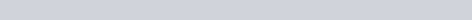It might seem strange that the n-value is specified. The reason we need the n-value to find the answer is that there are, in theory, an infinite number of molecular formulas that share the empirical formula C3H4N2, one for every value of n. Therefore, we need to know “where we’re going” beforehand.

## Finding molecular formulas from data

In general, the procedure for finding molecular formulas from experimental data will be as follows:

1. Write the moles of each element in the sample. These can be found from experimental data like reaction products, percent composition, or otherwise.
2. Find the element with the least moles. Divide the moles of every element by that amount. This will give the ratio of each element to the one with the least moles. These ratios may not be whole numbers, e.g. 1.33, 2.5, etc.
3. If the relative amounts are all whole numbers, skip this step. Otherwise, pick one number to multiply every relative amount of each element by so that they become whole numbers. For example, C = 1.67, H = 2.33, O = 1 would be transformed to C = 5, H = 8, O = 3 by multiplying through by 3.
4. Write a formula with subscripts equal to the numbers obtained in the last step. The example above would be written C5H8O3. You’re done! That’s the empirical formula.

## Practice Problem – An Empirical Formula Experiment

Below is an example of how one can find the molecular formula with experimental data by using the empirical formula.

### Setup

A chemist receives a canister of an unknown chemical, and she needs to figure out its molecular formula. She knows already that the chemical only contains carbon and hydrogen.

The researcher takes a 50.0 gram sample of the unknown compound and burns it in a combustion reaction with excess oxygen gas, creating CO2 and H2O. After collecting the products, she finds that the reaction produced 156.8 g CO2 and 64.2 g H2O.

### Finding the empirical formula

The chemist first finds the moles of each product: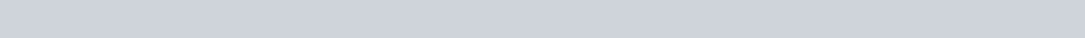Since all of the carbon in CO2 came from the original molecule, as did all of the hydrogen in water, the chemist further finds: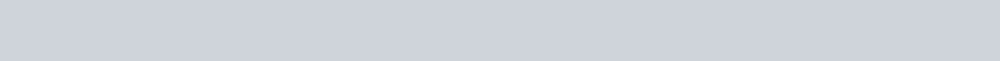There is one relative mole of carbon and two relative moles of hydrogen. This means that the empirical formula of the starting molecule is CH2.

### Finding the molecular formula

After some more testing, the chemist concludes that the molecular weight of the unknown chemical is 42.09 grams per mole. She finds the empirical weight (that is, the weight of the empirical formula of the compound, CH2) to be 14.03 grams per mole. By definition, the n-value times the empirical formula equals the molecular formula. She uses this relationship to find the following: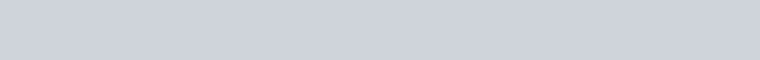With an n-value of 3, the chemist “multiplies” the empirical formula by 3 to find the molecular formula: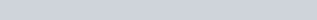The chemist therefore concludes that the molecular formula of the unknown compound is C3H6.

If you follow the steps in this tutorial, any empirical formula problem should be a breeze. Remember that many compounds will share the same empirical formula, and finding the differences based on mass, physical properties, reactivity, and other characteristics is key to discovering the molecular formula.